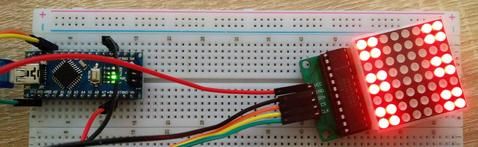Generating random numbers is easy. For this, there is the function random().

Let's try it with a small sketch that generates a number and outputs it on the serial monitor:

```Void Setup() {   Serial.Begin(9600);   Serial.Print("Random number between 1 and 6: ");   Serial.Print(Random(1,7));   Serial.Print("An");
}
Void Loop() {   put your main code here, to run repeatedly:
}  ```

We load the Sketch on our Nano, open the serial monitor, set 9600 Baud, and are happy:

Random number between 1 and 6: 2

If you press the reset button a couple of times it becomes obvious:

Random number between 1 and 6: 2
Random number between 1 and 6: 2
Random number between 1 and 6: 2
Random number between 1 and 6: 2
Random number between 1 and 6: 2

In the official documentation it is of course stated that one should initialize the random number generator by means of randomSeed() and recommends to read out a pin by analogRead() which is not connected.

It was only by chance that I came across sirleech's TrueRandom library. This provides a TrueRandom.random(function) that can be called like the random() function, but provides reliable random numbers without further preparation.

The library is available at GitHub at https://github.com/sirleech/TrueRandom

So we're adjusting our sketch a little bit:

```#include <TrueRandom.H> / Include the library
Void Setup() {   Serial.Begin(9600);   Serial.Print("Random number between 1 and 6: ");   Serial.Print(Random(1,7));   Serial.Print("An");   Serial.Print("Random number between 1 and 6 with TrueRandom: ");   Serial.Print(TrueRandom.Random(1,7));    Serial.Print("An");
}
Void Loop() {   put your main code here, to run repeatedly:
}

```

Also this sketch we load on our nano, and behold, a different number is now generated after each reboot:

Random number between 1 and 6: 2
Random number between 1 and 6 with TrueRandom: 2
Random number between 1 and 6: 2
Random number between 1 and 6 with TrueRandom: 1
Random number between 1 and 6: 2
Random number between 1 and 6 with TrueRandom: 4
Random number between 1 and 6: 2
Random number between 1 and 6 with TrueRandom: 2
Random number between 1 and 6: 2
Random number between 1 and 6 with TrueRandom: 6

TrueRandom has more to offer. Thus, the function TrueRandom.memfill(address, length) allows to fill a memory area at the specified address with random numbers.

It is also easy to generate MAC addresses or UUIDs.

We now include 64-man LED Matrix Displayto our nano, turn it into a cube.

First of all, we modify the June 7 blog post MD_MAX72xx_font.cpp as follows:

3, 0x42, 0x7f, 0x40, / 49 - '1'
5, 0x72, 0x49, 0x49, 0x49, 0x46, 50 - '2'
5, 0x21, 0x41, 0x49, 0x4d, 0x33, 51 - '3'
5, 0x18, 0x14, 0x12, 0x7f, 0x10, 52 - '4'
5, 0x27, 0x45, 0x45, 0x45, 0x39, / 53 - '5'
5, 0x3c, 0x4a, 0x49, 0x49, 0x31, 54 - '6'

replace with:

8.0x0,0x0,0x0,0x18,0x18,0x0,0x0,0x0, / 49 - '1'
8.0x3.0x3.0x0.0x18,0x18,0x0,0xC0,0xC0, 50 - '2'
8.0x3.0x3.0x0.0x18,0x18,0x0,0xC0,0xC0, 51 - '3'
8.0xC3,0xC3,0x0,0x0,0x0,0x0,0xC3,0xC3, 52 - '4'
8.0xC3.0xC3,0x0,0x18,0x18,0x0,0xC3,0xC3, / 53 - '5'
8.0xDB,0xDB,0x0,0x0,0x0,0x0,0xDB,0xDB, 54 - '6'

And finally, we include the MD_MAX72xx and MD_Parola libraries, and output the number on the matrix:

```#include <TrueRandom.H>
#include <MD_MAX72xx.H>
#include <MD_Parola.H>
#include <Spi.H>

#define  MAX_DEVICES 1
#define CLK_PIN   13  // or SCK
#define DATA_PIN  11  // or MOSI
#define CS_PIN    10  // or SS

// Hardware SPI connection
MD_Parola P = MD_Parola(CS_PIN, MAX_DEVICES);
#define  DELAYTIME  100  // in milliseconds

void setup() {   Serial.begin(9600);   Serial.print("Zufallszahl zwischen 1 und 6: ");   Serial.print(random(1,7));   Serial.print("\n");   Serial.print("Zufallszahl zwischen 1 und 6 mit TrueRandom: ");   uint8_t rnd = TrueRandom.random(1,7);   Serial.print(rnd);    Serial.print("\n");   P.begin();   P.print(" ");   delay(500);   P.print(rnd);    }

void loop() {   // put your main code here, to run repeatedly:

}

```

Nun wird bei jedem neustart (Reset-Taster drücken) eine neue Zahl generiert und angezeigt.1 commentPeter Nohle

Hallo. Ich habe den Sketch an 3 Nanos probiert. Bei allen leuchtet nur alle 64 LEDs der Matrix. Als blutiger Anfänger habe ich jetzt im Code nix auffälliges entdeckt. Nach dem Upload des Sketches der fehlerfrei verläuft, blinken nach einem Reset auch kurz zwei Leds
auf dem Nano. Das heißt er arbeitet das Setup ab. Nur… Die Matrix bringt keine Änderung. Alle leuchten. Die cpp hab ich angepaßt. Was mache ich falsch?